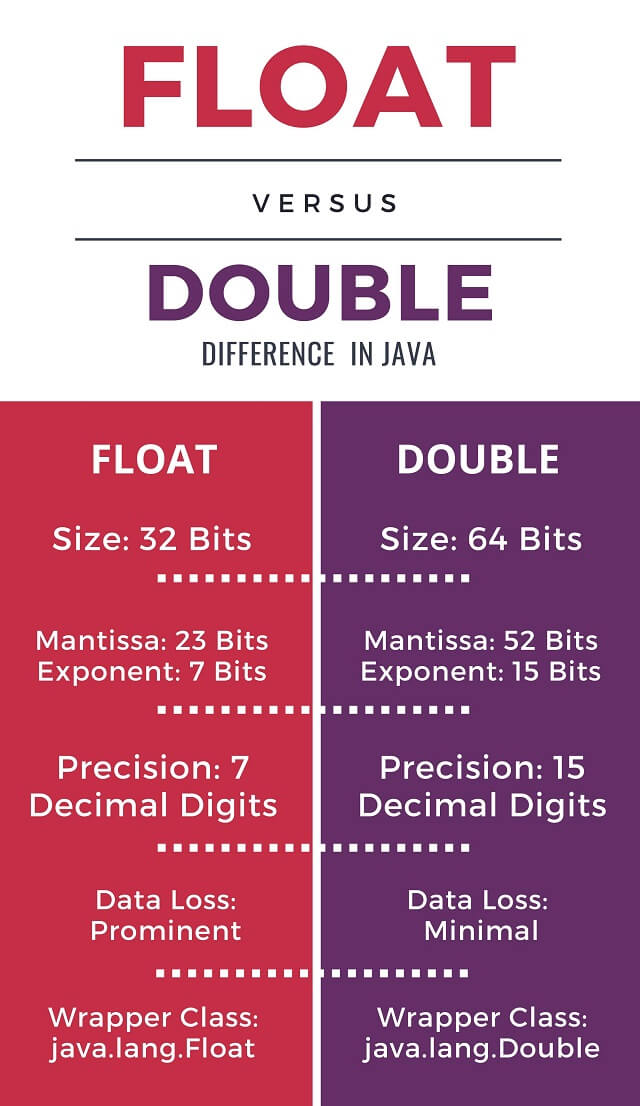# Java: Float vs Double | 4 Main Differences (& when to use?)

Every programming language deals with a wide range of data types. In Java, floating-point numbers are represented with the help of two data types: float and double. If you wish to dive deeper into the language of Java and know some of the important concepts of data types, then this article is definitely for you. Our article on the differences between float and double in Java should help you learn about the underlying concepts for the two data types. We also included a comparison table for float vs double in java programming.

## What is Float & Double?

Broadly, data can be divided into two main types: numeric and non-numeric data. Non-numeric data include characters and strings. Numeric data consists of whole numbers and floating-point numbers. Whole numbers, like the name, says, have only a whole part – there is no fraction involved. Floating-point numbers, on the other hand, come with a fractional part and a whole part out of the box. Depending on the size of the data required, whole numbers are assigned different data types that can be used depending on the requirement. Similarly, depending on the size of the number - especially the fractional part: floating-point numbers can also be assigned different data types. Float and Double are used to represent Floating-point numbers.

Float and double are the two data types Java allocates for dealing with floating-point numbers. Both data types are primitive by nature: meaning that their definitions are included as part of the language itself (or more specifically, the java.lang package – the default import for any Java program). Why exactly are two data types allocated for doing the same job? Well, they vary a lot in their scope and usability in various scenarios.

Let’s have a look at how different these two data types are.

## 4 Differences b/w Float and Double in Java

So, here are 4 main differences between float and double in Java:

1. Size: Float is of size 32 bits while double is of size 64 bits. Hence, double can handle much bigger fractional numbers than float. They differ in the allocation of bits for the representation of the number. Both float and double use 1 bit for representing the sign of the number. However, they differ in the allocation of bits for the mantissa and the exponent. Float allocates 23 bits for mantissa, while double allocates 52 bits for mantissa. Similarly, float uses 7 bits for exponent while double allocate 11 bits for exponent.
2. Precision: Float can allow only 7 bits of precision. Double allocates almost double the number of bits for the exponent – allowing it to handle up to 15 bits of precision. Having an extremely small exponent size means there is some amount of data compression while working with the float. Data compression inevitably means some of the bits are lost. This loss of bits from the end of the number is very pronounced while using float. The number of bits lost is much lesser while using double because of the large number of bits allocated for the exponent.
3. Default: Double is the default data type used by Java for dealing with fractional numbers. To force Java to use the float data type, the character ‘f’ or ‘F’ must be suffixed to the number. This can also be done by forcibly typecasting the number to float by prefixing (float) to the number at the time of initialization.
4. Wrapper Class: Both float and double are primitive types. This means that they will have their wrapper classes which allow them to be used as object types. The wrapper class for float is java.lang.Float while the wrapper class for double is java.lang.Double.

### Float vs Double in Java Comparison

The following infographic summarizes the differences between float and double in java:### When to use Float and Double in Java?

Float is generally used when speed is more important than accuracy. While our day-to-day programs do not involve large computations, in practical applications, this difference becomes quite significant. Also, the float is generally used when the number of decimal digits required is known. Double is used for almost all other cases: especially when precision is highly important. Keep in mind that Java enforces the use of double as the default data type for dealing with floating-point numbers.

## Conclusion

Float and double are two of the data types allocated by Java for dealing with floating-point numbers (or fractional numbers). While they might seem similar, in reality, they are quite different from each other. Do you need help with understanding java's float and double types? Our java online help experts are available. Our article on the key differences between these two data types summarizes Float vs Double in java programming.

### FavTutor - 24x7 Live Coding Help from Expert Tutors!